By going through these CBSE Class 11 Chemistry Notes Chapter 12 Organic Chemistry Some Basic Principles and Techniques, students can recall all the concepts quickly.

## Organic Chemistry Some Basic Principles and Techniques Notes Class 11 Chemistry Chapter 12

→ Carbon: Tetra valency of carbon, shape of organic compounds & characteristic features of π-bond.

→ Structural representation of organic compounds: Complete, condensed & bond line structural formulae.

→ A 3-dimensional representation of organic molecules & classification of organic compounds.

→ Acyclic or open chain compounds & Alicyclic or closed chain compounds or ring compounds & functional groups.

→ Homologous series, Nomenclature of organic compounds & I.U.P.A.C. nomenclature of alkanes.

→ Nomenclature of organic compounds having a functional group or groups & nomenclature of substituted benzene compounds.

→ Isomerism: Structural, chain, position, functional group isomerism, metamerism & stereoisomerism.

→ Fundamental concepts in organic reaction mechanism: Fission of a covalent bond, Nucleophiles & Electrophiles, Electron movement in organic reactions. Electron displacement effects in covalent bonds. Inductive effect, resonance structure & resonance effect.

→ Electromeric effect (E-effect), Hyperconjugation, types of organic reactions & mechanisms.

→ Methods of purification of organic compounds: Sublimation, crystallization, distillation, differential extraction, chromatography.

→ Qualitative analysis of organic compounds detection of C & H, N, S, halogens & for PO43-.

→ Quantitative analysis of organic compounds: Elemental detection in the form of a percentage, C, H, N-(Dumas method, Kjeldahl’s method), halogens, sulfur, phosphorus & oxygen.

→ Orbital hybridization concept: The nature of the covalent bonding in organic compounds can be described in terms of the orbitals hybridization concept.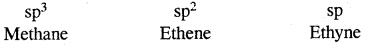→ Three-dimensional representation of organic compounds: Three-dimensional representation of organic compounds on paper can be drawn by wedge & dash formula.

→ Functional group: A functional group is an atom or group of atoms bonded together in a unique fashion & which determines the physical & chemical properties of compounds.

→ I.U.P.A.C.: International union of pure & applied chemistry.

→ Organic reaction mechanism: Organic reaction mechanism concepts are based on the structure of the substrate molecule. Fission of a covalent bond, the attacking reagents, the electron displacement effects & the conditions of the reaction.

→ Cleavage of covalent bond: A covalent bond may be cleaved in a heterolytic or homolytic fashion. A Heterolytic cleavage yields carbocations or carbanions & a homolytic cleavage gives free radicals as reactive intermediates.

→ Nucleophile & Electrophile:

• Nucleophile – Electron pair donor
• Electrophile – Electron pair acceptor.

→ Organic reactions:

1. Substitution reactions
3. Elimination reactions
4. Re-arrangement reactions

→ Methods of purifications of organic compounds:

1. Sublimation
2. Distillation &
3. Differential extraction

→ Chromatography is a useful technique of separation, identification & purification of compounds. It is classified into two categories adsorption & partition chromatography. Lassaigne’s Test: N, S, halogens & phosphorus are detected by Lassaigne’s test.

→ Estimation of C & H: Carbon & hydrogen are estimated by determining the amounts of CO2 & water produced. Estimation of Nitrogen: Nitrogen is estimated by Duma’s or Kjeldahl’s method.

→ Halogens Estimation: Halogens are estimated by various methods. Estimation of S & Phosphorus: S & P are estimated by oxidizing them to sulphuric & phosphoric acid respectively.

→ The percentage of oxygen: The percentage of oxygen is usually determined by subtracted (the sum of percentages of all other elements present in the compound) out of 100.

→ Retardation factor:
Rf = $$\frac{\text { Distance moved by the substance from base line }}{\text { Distance moved by solvent from base line }}$$
(a) Percentage of carbon = $$\frac{12 \times m_{1} \times 100}{44 \times m}$$
m1 = mass of CO2
m = mass of organic compound

(b) Percentage of Hydrogen = $$\frac{2 \times m_{1} \times 100}{18 \times m}$$
m1 = mass of H2O
m = mass of organic compound

(c) Percentage of nitrogen by Dumas method = $$\frac{28 \times V \times 100}{22400 \times m}$$
V = Volume of nitrogen m mass of organic compound

(d) Percentage of nitrogen by kJeldahl’s method = $$\frac{1.4 \times \mathrm{M} \times 2\left(\mathrm{~V}-\mathrm{V}_{1} / 2\right)}{m}$$
m mass of organic compound
M = Molarity of H2SO4 taken
V = Volume of H2SO4 of molarity-M
V1 = Volume of NaOH of molarity-M used for titration of excess of H2S04

(e) Percentage of halogens = $$\frac{\text { Atomic mass of }(\mathrm{X}) X m_{1} g \times 100}{\text { molecular mass of }(\mathrm{AgX}) X m}$$
m = mass of organic compound
m1 = mass of AgX formed
X = halogen atom

(f) Percentage of sulphur = $$\frac{32 \times m_{1} \times 100}{233 \times m}$$
m = mass of organic compound
m1 = mass of BaSO4 formed

(g) Percentage of Phosphorus:
If Phosphorus is estimated as Mg2P2O7
= $$\frac{62 \times m_{1} \times 100}{222 \times m}$$
m = mass of organic compound
m1 = mass of Mg2P2O7
222 = molar mass of Mg2P2O7

If Phosphorus is estimated as (NH4)3 PO4.12MoO3 then percentage of Phosphorus = $$\frac{31 \times m_{1} \times 100}{1877 \times m}$$
here m1 = mass of (NH4)3 PO4.12MoO3
1877 = molar mass of (NH4)3PO4.12MoO3

(h) Percentage of Oxygen:
= $$\frac{32 \times m_{1} \times 100}{44 \times m}$$
m = mass of organic compound
m1 = mass of carbondioxide

Chapter In Brief:
Berzelius, A Swedish chemist proposed that a Vital Force was responsible for the formation of organic compounds. F. Wohler gave a death blow to the Vital Force theory when he synthesized organic compound urea from an inorganic compound ammonium cyanate.Tetravalence of Carbon: Shapes of organic compounds: The formation of CH4, C2H6 is due to sp3 hybridization of C; formation of CH4 is on the basis of sp2 hybridization of C, and formation of C2H2 is on the basis of sp hybridization of C. The presence of double bond in H2C=CH2 and triple bond in HC = CH is due to the presence of one π and two π bonds respectively in them.

In H2C=CH2, rotation about C-C bond is hindered due to the presence of π bond between the two C atoms, sp3 hybridization gives rise to tetrahedral shape t

o CH4, sp2 hybridization gives rise to a trigonal planar arrangement to C2H4, and sp hybridization gives linear shape to C2H2. An sp3 hybrid orbital can overlap with Is orbital of hydrogen to give a C—H bond (sigma a single bond). Overlap of an sp2 orbital of one carbon with an sp2 orbital of another results in the formation of a carbon-carbon bond.

The unhybridized p-orbitals on two adjacent carbons can undergo lateral (side-by-side) overlap to give a pi (π) bond. Organic compounds can be represented by various structural formulas. The three-dimensional representation of organic compounds on paper can be drawn by the wedge and dash formula.

Organic compounds can be classified on the basis of their structure or the functional groups they contain. A functional group is an atom or group of atoms bonded together in a unique fashion which determines the physical and chemical properties of the compounds. The naming of the organic compounds is carried out by following a set of rules laid down by the International Union of Pure and Applied Chemistry (IUPAC). In IUPAC nomenclature, the names are correlated with the structure in such a way that the reader can deduce the structure from the name.

Structural Representations Of Organic Compounds:
Complete, condensed, and Bond-line structural formulae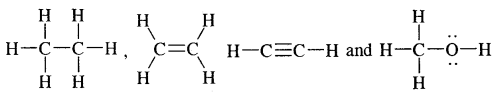stand for the complete structural formulae of ethane, ethene ethyne, and methanol whereas CH3—CH33 (or C2H6), H2C=CH2 (or C2H4), HC ≡ CH (or C2H2), and CH3OH stand for their condensed structural formulae respectively.

Bond-line structural representation of 1,3 butadiene is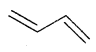and that of 3-methyl octane is(its condensed formula is CH3CH2CH(CH3) (CH2)4CH3

The bond-line structure of chlorocyclohexane is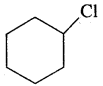Three Dimensional Representation Of Organic Molecules:
The three-dimensional (3-D) structure of organic molecules can be represented on paper by using certain conventions.

For example by using solid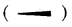and dashedwedge formula, the 3-D image of a molecule from a two-dimensional picture can be perceived. The solid wedge projects towards the observer and the dashed wedge projects away from the observer. The bonds lying in the plane of the paper are depicted by using a normal line (—)

The 3-D representation of CH4 is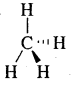Classification of Organic Compounds:1. Acyclic or Open Chain Compounds: These compounds are also called aliphatic compounds and consist of straight or branched chain compounds.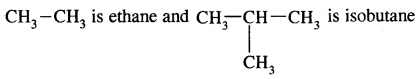is acetaldehyde and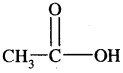is acetic acid.

2. Alicyclic or Closed Chain or Ring Compounds:
Some of the examples of alicyclic /closed chain or ring compounds are as follows:Aromatic Compounds: Benzenoid Aromatic Compounds:Non-Benzenoid CompoundsHetero Cyclic Aromatic Compounds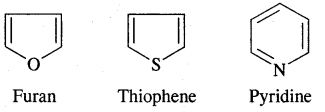→ Functional Group:
The functional group may be defined as an atom or group of atoms joined in a specific manner that is responsible for the characteristic chemical properties of the organic compounds. The examples are hydroxyl group (-OH), aldehyde group (-CHO) and carboxylic acid group (—COOH), etc.

→ Homologous Series:
A group or a series of organic compounds each containing a characteristic functional group forms a homologous series and the member of the series are called homologs. The members of a homologous series can be represented by general molecular formula and the successive members differ from each other in the molecular formula by a — CH2 unit. There are a number of homologous series of organic compounds. Some of these are alkanes, alkenes, alkynes, alkyl halides, alkanols, alkanols, alkenones, alkanoic acids, amines, etc.

e.g. The general formula of alkanols is CnH2n+1-OH. Individual members of a homologous series are called Homologues.
CH3OH, C2H5OH, C3H7OH are homologs of the alkanol family.

→ Nomenclature of Organic Compounds:
Earlier organic compounds were known by their common or trivial names. For example, HCOOH was called formic acid, CH3 CHO was called acetaldehyde, and so on.

→ The I.U.P.A.C System Of Nomenclature:
To systematize the naming of millions of organic compounds IUPAC (International Union Of Pure And Applied Chemistry) pattern of naming is adopted.

The I.U.P.A.C. System Of Nomenclature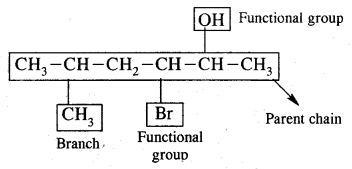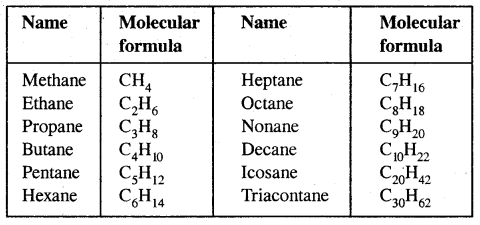Common or Trivial names of some organic compounds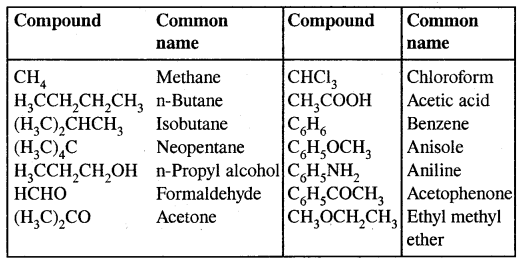Table: Some functional Groups and classes of organic compounds: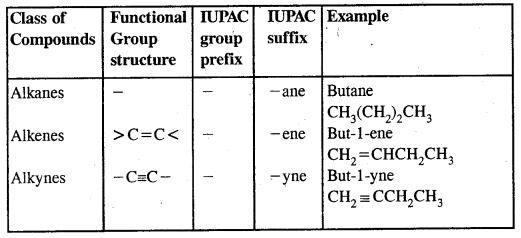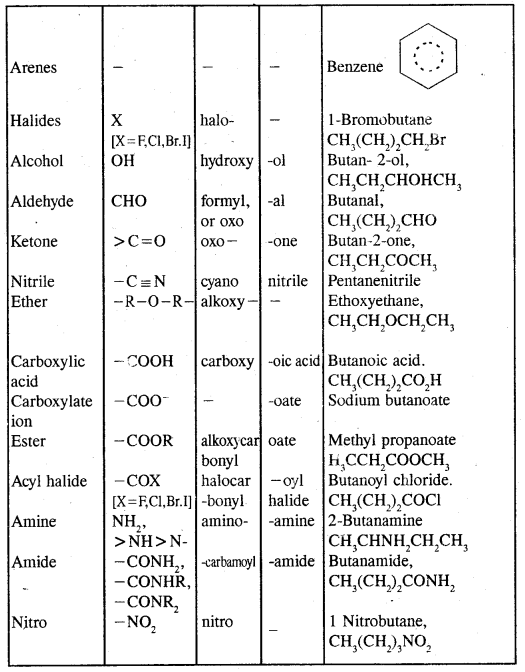Note: Students are advised to follow different rules and conventions as per the IUPAC system as given in the Textbook. In the case of polyfunctional compounds, one of the functional groups is chosen as the principal functional group and the compound is named on that basis.

The remaining functional groups which are subordinate functional groups are named as substituents using the appropriate prefixes. The choice of the principal functional group is made on the basis of the order of preference. The order of decreasing priority for the same functional groups is:
-COOH, -SO3H, -COOR (R = alkyl group)
-COCl, -CONH2, -C ≡ N, -CHO, > C = O, -OH, -NH2,The R, C6H5, halogens (F, Cl, Br, I), NO2, alkoxy (OR), etc. are always prefixed substituents.

For example:
(i) HOCH2(CH2)3CH2COCH3 will be named as 7 hydroxyheptan- 2-one
(ii) Br CH2CH = CH2 is named as 3-Bromoprop-l-ene.
(iii) CH2 = CH-CH = CH2 is Buta-1, 3-diene.Problem:
Derive the structure of
1. 2-chioropentane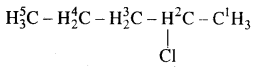2. Pent-4-en-2-ol3. 3-Nitrocyclohexene4. Cyclohex-2-en-l-ol5. 6-Hydroxyheptanal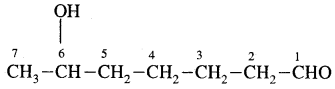Nomenclature of Substituted Benzene Compounds: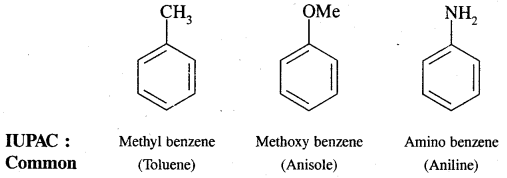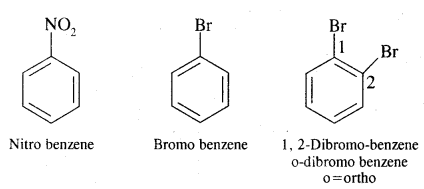Problem: Write the structural formula of
(a) o-Ethyl anisole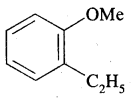(b) p-Nitroaniline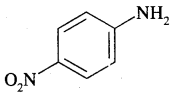(c) 2, 3-dibromo-l-phenyl pentane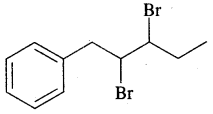(d) 4-Ethyl-l-fluoro-2-nitrobenzeneIsomerism: The phenomenon of the existence of two or more compounds possessing the same molecularformula but different properties is known as isomerism. Such compounds are called isomers. The above flow chart shows different types of isomerism.

Types of structural isomerism:
1. Chain isomerism: This type of isomerism is due to the difference in the nature of the carbon chain (i.e., straight or branched) which forms the nucleus of the molecule, e.g.,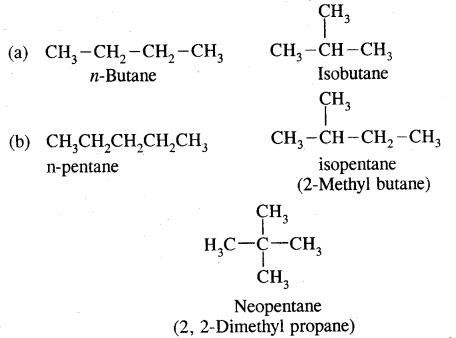2. Position isomerism: It is due to the difference in the position of the substituent atom or group or an unsaturated linkage in the same carbon chain. Examples are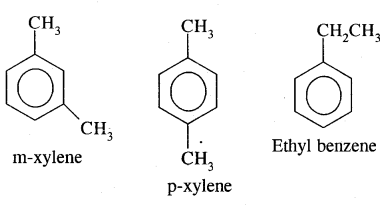3. Functional isomerism: Two or more compounds having the same molecular formula but different functional groups are called functional isomers and this phenomenon is termed functional group isomerism. For example, the molecular formula C3H6O represents an aldehyde and a ketone.and C3H6O represents an ether and alcohol.4. Metamerism: It is due to the difference in nature of the alkyl group attached to the same functional group. This type of isomerism is shown by compounds of the same homologous series.
For example.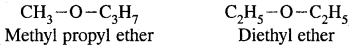II. Stereoisomers: Stereoisomers are compounds that have the same constitution and sequence of covalent bonds but differ in the relative positions of their atoms or groups in space.

5. Geometrical isomerism: The isomers which possess the same structural formula but differ in the spatial arrangement of the groups around the double bond are known as geometrical isomers and the phenomenon is known as geometrical isomerism. This Isomerism is shown by alkenes or their derivatives. When the similar groups lie on the same side, it is the cis-isomer, while when the similar groups lie on opposite sides, the isomer is trans. For example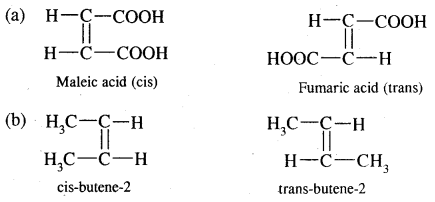Fundamental Concepts In Organic Reaction Mechanism:
In an organic reaction, the organic molecules (substrate) reacts with an appropriate attacking reagent and leads to the formation of one or more intermediates and finally product (s)

The general reaction is depicted as follows: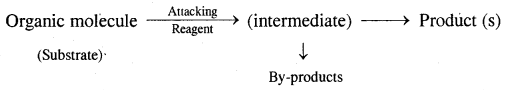The substrate is that reactant which supplies carbon to the new bond and the other reactant is called reagent. A sequential account of each step, details of electron movement, energetics during bond breaking and bond formation, and the details of timing, when a reactant is transformed into the product are referred to as Reaction Mechanism.

Fission of a Covalent Bond: It occurs in two ways.
(A) Homolytical Fission/Cleavage or Homolysis
In such fission, each atom gets one electron of the shared pair of electrons.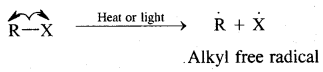Alkyl radicals are classified as primary secondary or tertiary. Alkyl radical stability increases as we proceed from primary to tertiary. Organic reactions, which proceed by homolytic fission are called free radical or homopolar, or non-polar reactions.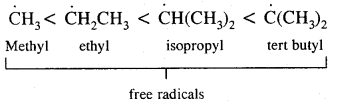→ Heterolytical Fission/Cleavage or Heterolysis: The covalent bond breaks in such a way that the shared pair of electrons remains with one of the fragments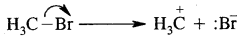The species that has a sextet at the carbon and is positively charged is called a Carbocation (or carbonium ion)
The shape of methyl carbocation C is sp2 hybridized and its shape is Trigonal PlanarThe observed order of carbocation stability isThe fission can occur, the other way.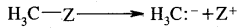: -CH3 is called a Carbanion. Such a carbon species carrying a negative charge is called a Carbanion. Their stability decreases as follows: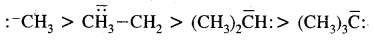Nucleophiles & Electrophiles:
(A) Nucleophile (Nu:): A reagent that is electron-rich and is in search of a relatively positive center is called a nucleophile. Example of nucleophiles are

Negatively charged reagents: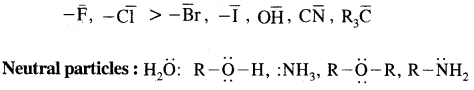(B) Electrophiles: A recent which is electron-deficient and is in search of electron-rick site is called an electrophile Positively charged electrophiles are: H+, H3O+, NO2+, R+, Br4
Neutral particles: BF3, AlCl3, SO3

Inductive Effect (I effect):
It is the process of displacement of electrons along the chain of carbon atoms due to the presence of a polar covalent bond at one end of the chain. This is a permanent effect. It is of two types:
(A) -I effect: When the atom or group of atoms of the polar covalent bond is more electronegative than C, it is said to show the -I effect.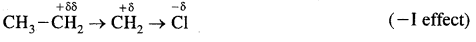It is practically over after C2
The —I effect of some of the atoms or groups of atoms in decreasing order is
-NO2 > -CN > -COOH > -F > -Cl > -Br > -I

(B) + I effect: If the substituent attached to the end of the carbon chain is electron-donating, the effect is called + I effect.The + I effect of some of the atoms or groups of atoms in the decreasing order is→ Electromeric Effect (E-effect): It involves the complete- transfer of electrons of multiple bonds (double or triple bond) to one of the bonded atoms (usually more electronegative) at the call of the attacking reagent. It vanishes the moment the attacking reagent is removed. It is a temporary effect.

It is also of two types – E and + E effect.
If the electrons of the bond are transferred to that atom of the double bond to which the reagent finally gets attached the effect is called the + E effect.If the electrons of the double bond are transferred to an atom of the double bond other than the one to which the reagent gets finally attached, the effect is called the — E effect.→ Resonance Or Mesomerism: The phenomenon of resonance is said to occur whenever for a molecule we can write two or more Lewis structures that differ in the positions of electrons but not in the relative position of atoms. The various Lewis structures are called responding/canonical/contributing structures. The actual structure of the molecule is not represented by any of the resonance structures but is a resonance hybrid of all these canonical structures.

The various resonance structures are separated by a double-headed arrow ↔ Benzene is a resonance hybrid of the two Kekule structures.Any of the two structures cannot explain all the properties of benzene. But the resonance hybrid which cannot be drawn on the paper and which is the actual structure of benzene will explain all the properties of benzene. For example, there are 3 double bonds and 3 single bonds (3 C = C and 3 C — C) in benzene corresponding to bond lengths of 1.34 Å and 1.54 Å respectively.

But as X-ray diffraction studies point out there are no single or double bonds in benzene and all the C—C bonds are having a bond length of 1.39 Å and are exactly equivalent. The resonance hybrid of benzene is generally shown by III.

Another example of resonance is provided by CH3NO2 (nitromethane).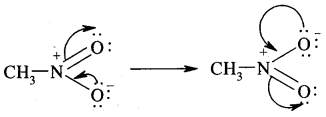Hyper Conjugation:
It is regarded as no bond resonance. Hyperconjugation is a general stabilizing interaction. It involves delocalization of an electron of C-H bond of an alkyl group directly attached to an atom of the unsaturated system; or to an atom with an unshared p orbital. The electrons of C—H a bond of the alkyl group enter into partial conjugation with the attached unsaturated system or with the unshared p orbital. Hyperconjugation is a permanent effect.

To understand the hyperconjugation effect, let us take an example of CH3+CH2 (ethyl cation) in which the positively charged carbon atom has an empty n orbital. One of the C-H CT bonds of the methyl group can align in the plane of this empty n orbital and the electrons constituting the C—H bond in-plane with this π orbital can then be delocalized into the empty π orbital as depicted in Fig.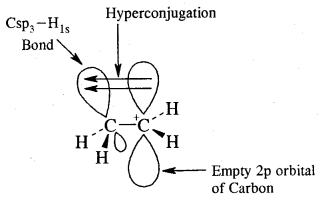Orbital diagram showing hyperconjugation in ethyl cation

This type of overlap stabilizes the carbocation because electron density from the adjacent bond helps in dispersing the positive charge. In general, the greater the number of alkyl groups attached to a positively charged carbon atom, the greater is the hyperconjugation interaction and stabilization of the cation. Thus, we have the following relative stability of carbocations:Hyperconjugation is also possible in alkenes and alkyl arenes. Delocalisation of electrons by hyperconjugation in the case of an alkene can be depicted as in Fig.(b)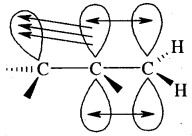Orbital diagram showing hyperconjugation in propene

There are various ways of looking at the hyperconjugation effect. One of the ways is to regard the C-H bond as possessing partial ionic character due to resonance.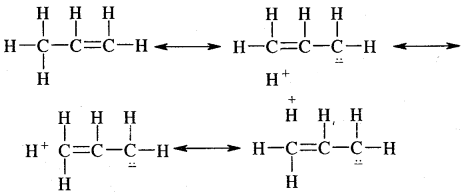Problem: Explain why (CH3)3C+ is more stable than CH3 CH2+ and CH3+ is the least stable cation.
Answer: Hyperconjugation interaction in (CH3)3C+ is greater than in CH3CH2+ as the (CH3)3C+ has nine C—H bonds. In CH3, vacant n orbital is perpendicular to the plane in which C-H bonds lie, hence cannot overlap with it. Thus CH3+ lacks hyper conjugative stability.

Types Of Organic Reactions:
1. Substitution Reactions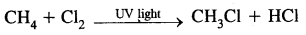H2C = CH2 + HBr → CH3 – CH2Br

3. Elimination reactions
CH3—CHBr—CH3 + KOH → CH3-CH = CH2 + KBr + H2O

4. Rearrangement ReactionsMethods Of Purification Of Organic Compounds:
The common techniques used for the purification of organic compounds are based on their nature and the impurity present in them. The methods are as follows.

1. Sublimation
2. Crystallization
3. Distillation
4. Differential extraction
5. Chromatography

Finally, the purity of a compound is ascertained by determining its melting point or boiling point. Most of the pure compounds have sharp melting points and boiling points.

1. Sublimation: Some solid substances like camphor, naphthalene, etc. on heating change from solid to vapor state without passing through the liquid phase. The purification technique based on the above principle is known as sublimation and is used to separate sublimable compounds like benzoic acid from non-sublimable compounds like sodium chloride.

2. Crystallisation: It is based on the difference in the solubilities of the compound and the impurities in a suitable solvent. The impure compound is dissolved in a solvent in which it is sparingly soluble at room temperature but appreciably soluble at a higher temperature. The solution is concentrated by heating to get a nearly saturated solution. On cooling, crystals of the pure substance are removed by filtration.

3. Distillation: The process of distillation is carried out to separate

• volatile liquids from non-volatile impurities and
• liquids having sufficient differences in their boiling points.

Liquids having different boiling points vaporize at different temperatures. The vapors are cooled and get condensed into liquids. They are collected separately. CHCl3 (b.p. 334 K) and aniline (b.p. 457 K) are easily separated by this method.

Fractional Distillation:
It is resorted to when the difference in boiling points of two liquids is not much. It is carried out through an a.fractionating column fitted over the mouth of the round bottom flask.

One of the technological applications of fractional distillation is to separate different fractions of crude oil in the petroleum industry.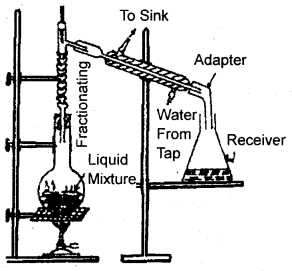Fractional. Distillation

The vapors of the less volatile liquid condense into the liquid which returns to the flask. The more volatile fraction passes over to the other side, condenses in the water condenser, and is collected in the receiver. When one fraction is completely separated the temperature is raised and the receiver is changed. Now, the second less volatile fraction distills over. Thus the more volatile liquid distills afterward. This is highly successful if the difference in b.p. of two liquids is less than 10-15 K.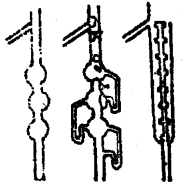Fractional. Distillation

Distillation Under Reduced Pressure:
This method is applicable to purify liquids having very high boiling points and those, which decompose at or below their boiling points. Such liquid is are made to boil at a temperature lower than their normal boiling points by reducing the pressure on their surface. A liquid boils at a temperature at which its vapor pressure becomes equal to the external pressure. The flowsheet diagram for distillation under reduced pressure is shown below.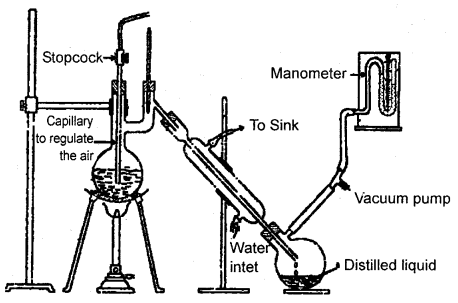Distillation under reduced pressure. A liquid boils at a temperature below its vapor pressure by reducing the pressure.

Steam Distillation:
This technique is applied to separate substances, which are steam, volatile, and are immiscible with water. In steam distillation, the steam generator is passed through a heated flask containing the liquid to be distilled. The mixture of steam and the volatile liquid is condensed and collected.

In steam distillation the liquid boils when the sum of the vapor pressures due to the organic liquid (p1) and that due to water (p2) become equal to the atmospheric pressure (p) i.e., p = p1 + p2 since p1 is lower than p, the organic liquid vapourised at a lower temperature than its b. pt. Thus if one of the substances in water and the other a water-insoluble substance such a mixture will boil close to but below 373 K. Aniline is separated from the aniline-water mixture by this method.Steam distillation. Steam volatile component volatilizes, the vapors condense in the condenser and the liquid collects in a conical flask.

Differential Extraction:
When an organic compound is present in an aqueous medium, it is separated by shaking with an organic solvent in which is more soluble than water. The organic solvent and the aqueous solution should be immiscible with each other so that they form two distinct layers which can be separated by the separatory funnel. The organic solvent is later removed by distillation or by evaporation to get back the compound.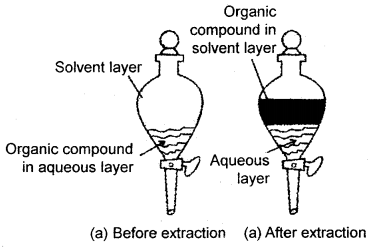(a) Differential extraction. Extraction of the compound takes place based on the difference in solubility

Chromatography:
Chromatography is an important technique extensively used to separate mixtures into their components, purify compounds, and also test the purity of compounds. In this technique, the mixture of substances is applied into a stationary phase, which may be a solid or a liquid.

A pure solvent, a mixture of solvents, or a gas is allowed to move slowly over the stationary phase. The components of the mixture get gradually separated from one another. The moving phase is called the mobile phase.

Based on the principle involved, chromatography is classified into different categories. Two of these are:
(b) Partition chromatography,

(a) Adsorption Chromatography: Adsorption chromatography is based on the fact that different compounds are adsorbed on an adsorbent to different degrees. Commonly used adsorbents are silica gel and alumina. When a mobile phase is allowed to move over a stationary phase (adsorbent), the components of the mixture move by varying distances over the stationary phase.

Following are two main types of chromatography techniques based on the principle of differentials adsorption.
(a) Column chromatography and
(b) Thin layer chromatography

(a) Column Chromatography: Column chromatography involves the separation of a mixture over a column of adsorbent (stationary phase) packed in a glass tube. The mixture adsorbed on the adsorbent is placed on top of the adsorbent in the column. An appropriate element which is a liquid or a mixture of liquid is allowed to flow down the column slowly. Depending upon the degree to which the compounds are adsorbed, complete separation takes place. The most readily adsorbed substances are retained near the top and the others come down to various distances in the column.Column chromatography. Different stages of separation of components of a mixture

(b) Thin Layer Chromatography (TLC): Another type of adsorption chromatography, which involves the separation, of substances of a mixture over a thin layer of an adsorbent coated on a glass plate. A thin layer of an adsorbent (silica or alumina SiO2 or Al2O3 gel) is spread over a glass plate. The solution of the mixture to be separated is applied as a small spot about 2 cm above one end of the TLC plate. The glass plate is then placed in a closed jar containing the eluent (see fig. below).

As the eluent rises up the plate the components of the mixture move up along with the eluent to different distances depending on their degree of adsorption and separation takes place. The relative adsorption of each component is expressed in terms of its Retention Factor, i.e., Rf Value
Rf = $$\frac{\text { Distance moved by the substance from baseline }(x)}{\text { Distance moved by the solvent from baseline }(y)}$$(a) Thin layer chromatography,
(b) Developed chromatogram. Chromatogram being developed

The spots of colored compounds are visible on the TLC plate due to their original color. Fig. (b) on the previous page.

Partition Chromatography:
Paper chromatography is a type of partition chromatography. Water trapped in chromatography paper acts as a stationary phase. It is based on the principle of continuous differential partitioning of components of a mixture between stationary and mobile phases. A strip of paper spotted at the base with the solution of the mixture is suspended in a suitable solvent.

The solvent acts as the mobile phase rise up due to capillary action and flows over the spot. The paper selectively retains different components according to their differing partition in two phases. The paper strip so developed is called Chromatogram. The spots of the separated colored compounds are visible at different heights from the position of the initial spot on the chromatogram. The spots may be observed under U. V. light as discussed in TLC.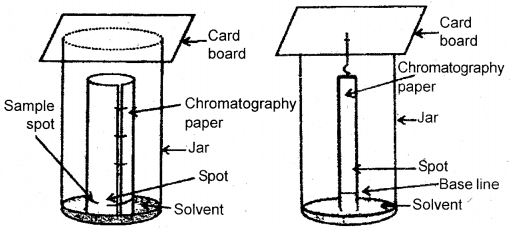Paper chromatography. Chromatography paper in two different shapes.

Qualitative Analysis Of Organic Compounds Detection Of Elements:
The elements present in the organic compound can be detected as follows:
1. Carbon and hydrogen: The given organic compound is mixed with about double the amount of pure and dry copper oxide. The mixture is heated in a hard glass tube. The CO2 and H2O produced due to combustion are tested by lime water and anhydrous copper sulfate. The lime water will turn milky and copper sulfate will turn blue.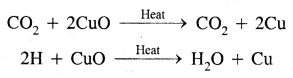2. Nitrogen, can be detected as
1. Soda-lime test: When the organic compound is heated with soda lime in a test tube, the evolution of ammonia indicates nitrogen.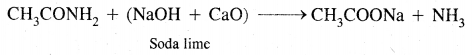2. Lassaigne’s test: A small piece of dry sodium is heated gently in a fusion tube till it melts to a shining globule. Then a small amount of organic substance is added and the tube is heated to red hot. The red hot tube is plunged into distilled water contained in a china dish. The contents of the dish are boiled, cooled, and filtered. The filtrate is known as sodium extract or Lassaigne’s extract.

For the nitrogen test, the sodium extract is made alkaline with a few drops of dil. NaOH. Freshly prepared FeSO4 solution is added and the contents are warmed. Then a few drops of FeCl3 are added followed by acidification with cone. HCl or H2SO4. The appearance of bluish-green coloration indicates nitrogen.If the organic compound contains N and S together, sodium thiocyanate (Na CNS) may be formed with the sodium extract which gives blood-red coloration due to the formation of Fe(CNS)3,

Sulfur: To the sodium extract.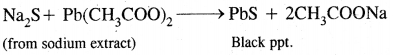2. To the other part of sodium extract add a few drops of sodium nitroprusside solution. The appearance of purple color indicates sulfur.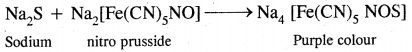Halogens:
This test can also be done with sodium extract. The extract is boiled with a cone. HNO3 to expel the gases. It is then cooled and treated with silver nitrate solution. The formation of different colored precipitates confirms halogens.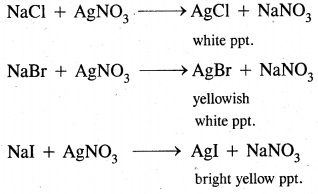Quantitative Analysis:
1. Estimation of Carbon and Hydrogen: A known weight of the organic compound is heated with dry cupric oxide in the dry atmosphere free from CO2. The carbon and hydrogen present, in the organic compound, are oxidized to CO2 and water. The CO2 is absorbed in potash bulbs and water is absorbed in CaCl2 tubes. From the weights of CO2, and H2O form, the percentage of C and H are calculated as: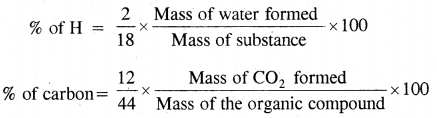2. Estimation Of Nitrogen: Nitrogen can be estimated by one of the following two methods.
1. Duma’s Method: A known weight of the given organic compound is heated with dry cupric oxide in a current of CO2. The N0 gas obtained is connected in Scliffs nitrometer at the prevailing temperature and pressure. Then, this volume of N, gas so collected is converted to volume at STP/NTP by using gas equation
P1V1/T1 = P2V2/T2
knowing 22.4 L of N2 gas at STP weight = 28.0 gm.

Weight and percentage of Nitrogen can be calculated
% of N = $$\frac{28}{22400} \times \frac{\text { Volume of } \mathrm{N} \text { at } \mathrm{STP} \text { in } \mathrm{mL}}{\text { Mass of compound }}$$ × 100

2. Kjeldahl’s Method: This is a more convenient method for the estimation of N particularly in foods, fertilizers, drugs etc. This method is, however not applicable to compounds containing nitrogen in the ring (Pyridine, quinoline, etc.) and compounds containing N directly linked to an oxygen atom (eg. NO2) or another N atom. e.g. A Z O (—N = N—) compounds.

In this method, the given organic compound is treated with a cone. H2SO4 to couvert N into (NH4)2 SO4 the ammonium sulfate [(NH4)2SO4 is treated with 40% NaOH solution and the ammonia evolved is neutralized with an excess of a standard acid [known volume V of the acid taken. The excess of the residual acid is titrated with a standard solution of the alkali and the volume of the acid left unneutralized by ammonia (v ml) is noted.

∴ Volume of the acid neutraL ised by ammonia = (V – u) ml.
∴ % of N = $$\frac{1.4 \times N(V+v)}{W}$$
where N = Normality of acid taken
W = wt. of the organic compound.

Estimation Of Halogens: The given organic compound containing halogens is treated in Carius Method with fuming nitric acid in a long-necked Carius Tube and silver nitrate. The halogen present is converted into silver halide. From the weights of silver halide formed and the known weight of the organic compound taken, the percentage of halogen can be calculated.

% of halogen = $$\frac{\text { Atomic mass of halogen }}{108+\text { At. mass of halogen }}$$ × $$\frac{\text { Mass of silver halide }}{\text { Mass of substance }}$$ × 100

Estimation of Sulphur:
In the Carius method organic compound is treated with fuming HNO3 and S is precipitated as BaSO4 by the addition of BaCl2. From the wt. of BaS04 formed, the percentage of S can be calculated.
% of sulphur = $$\frac{32}{233} \times \frac{\text { Mass of } \mathrm{BaSO}_{4}}{\text { Mass of substance }}$$ × 100

Estimation of Phosphorus:
In Carius method, P is quantitatively oxidized to H3PO4 by fuming HNO3 which is precipitated to Mg2P2O7. knowing the wt. of Mg2P2O7 and that of the organic compound, percentage of P can be determined
% of P = $$\frac{62}{222} \times \frac{\text { Mass of } \mathrm{Mg}_{2} \mathrm{P}_{2} \mathrm{O}_{7} \text { formed }}{\text { Mass of the substance }}$$ × 100

Estimation of Oxygen:
There is no direct method available to estimate oxygen in the organic compound. The percentage of oxygen is usually found by subtracting the sum of the percentages of all elements present in the compound from 100.